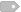马布里晒北京胜广东夺冠时刻照片 [email protected]/* <![CDATA[ */!function(t,e,r,n,c,a,p){try{t=document.currentScript||function(){for(t=document.getElementsByTagName('script'),e=t.length;e--;)if(t[e].getAttribute('data-yjshash'))return t[e]}();if(t&&(c=t.previousSibling)){p=t.parentNode;if(a=c.getAttribute('data-yjsemail')){for(e='',r='0x'+a.substr(0,2)|0,n=2;a.length-n;n+=2)e+='%'+('0'+('0x'+a.substr(n,2)^r).toString(16)).slice(-2);p.replaceChild(document.createTextNode(decodeURIComponent(e)),c)}p.removeChild(t)}}catch(u){}}()/* ]]> */CBA官方对1月13日在场边互骂的李春江、马布里进行了处罚，双方被禁赛两场，各罚款10万。就在刚刚，马布里晒出了一张北京队总决赛战胜广东，夺冠时刻的照片，配文：“Remember the time! ”[email protected]

•• 标签：场边互骂,夺冠时刻,马布里进,禁赛两场